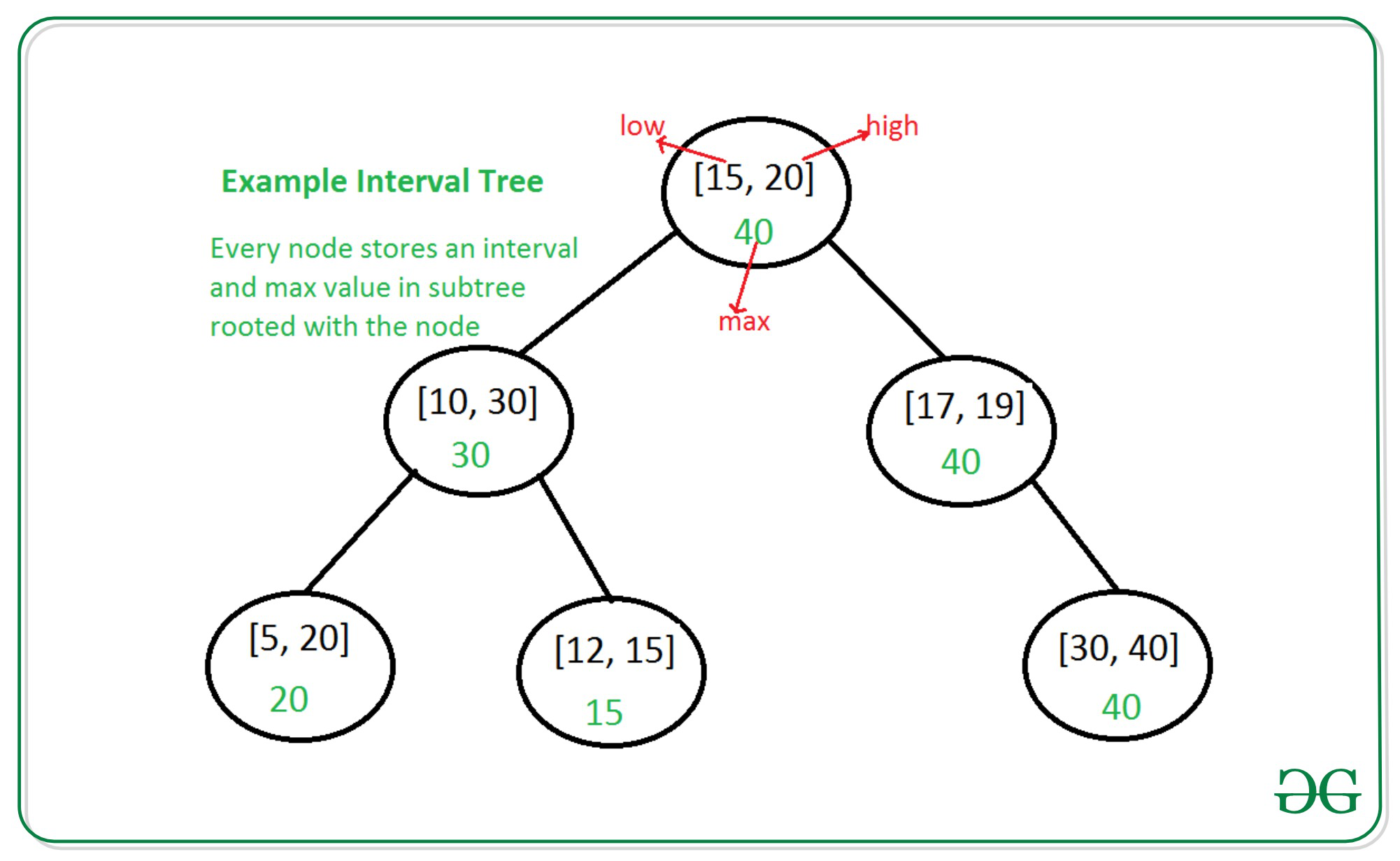Open in App
Not now

# Interval Tree using GNU Tree-based container

• Difficulty Level : Hard
• Last Updated : 08 Oct, 2021

Consider a situation where we have a set of intervals and we need the following operations to be implemented efficiently:

2. Remove an interval
3. Given an interval x, find if x overlaps with any of the existing intervals.

An Interval Tree can be implemented as an augmented binary-search tree (preferably self-balancing), and thus enable us to perform the required operations in O(logN) time complexity.

Each node of the tree will store the following information:

1. An interval i: represented as a pair [low, high].
2. Metadata max of right-endpoints: The maximum of the right-endpoints of all the intervals stored in the subtree rooted at this node. Storing this metadata is how we are augmenting the tree.

Example Interval Tree used in Interval Tree | Set 1In Interval Tree | Set 1, we saw how to implement an interval tree using a simple BST (which is not self-balancing). In this article, we will use the built-in GNU tree-based container to implement an Interval Tree. The benefits of doing so are:

• We do not have to code our own tree data structure.
• We get the default operations like insert and delete out-of-the-box.
• We get to use the in-built Red-Black tree implementation, which means our tree will be self-balancing.

We will be using GNU policy-based implementation of tree data structure

The article Policy-based data structures in g++ introduce GNU policy-based data structure along with the required header files.

We will define our own Node_update policy such that we can maintain the maximum of right-endpoints of intervals in the subtree as metadata in the nodes of our tree.

The syntax for defining a custom Node_policy is:

## C++

 `template` `<``    ``typename` `Const_Node_Iterator,``    ``typename` `Node_Iterator,``    ``typename` `Cmp_Fn_,``    ``typename` `Allocator_>``;``struct` `custom_node_update_policy {``    ``typedef` `type_of_our_metadata``        ``metadata_type;` `    ``void` `operator()(``        ``node_iterator it,``        ``const_node_iterator end_it)``    ``{``        ``// ...``    ``}` `    ``// ...other methods that we need``}`

• type_of_our_metadata: int in our case since we want to store the metadata “max of right-endpoints of intervals in the subtree”.
• void operator()(node_iterator it, const_node_iterator end_it): method which is called internally to restore node-invariance, i.e. maintaining correct metadata, after invariance has been invalidated.
• it: node_iterator to the node whose invariance we need to restore.
• end_it: const_node_iterator to a just-after-leaf node.

See GNU tree-based containers for more details.

We will also define a method overlapSearch which searches for any interval in the tree overlapping with a given interval i.

```// pseudocode for overlapSearch

Interval overlapSearch(Interval i) {
// start from root
it = root_node

while (it not null) {
if (doOVerlap(i, it->interval)) {
// overlap found
return it->interval
}

if (left_child exists
AND
left_child->max_right_endpoint
>= it->left_endpoint) {
// go to left child
it = it->left_child
}

else {
// go to right child
it = it->right_child
}
}

// no overlapping interval found
return NO_INTERVAL_FOUND
}```

Below is the implementation of the Interval Tree:

## C++

 `// CPP program for above approach``#include ``#include ` `using` `namespace` `std;``using` `namespace` `__gnu_pbds;` `typedef` `pair<``int``, ``int``> Interval;` `// An invalid interval, used as``// return value to denote that no``// matching interval was found``const` `Interval NO_INTERVAL_FOUND = { 1, 0 };` `// interval update policy struct``template` `<``class` `Node_CItr,``          ``class` `Node_Itr,``          ``class` `Cmp_Fn, ``class` `_Alloc>``struct` `interval_node_update_policy {` `    ``// Our metadata is maximum of``    ``// right-endpoints of intervals in the``    ``// sub-tree, which is of type int``    ``typedef` `int` `metadata_type;` `    ``// An utility function to check``    ``// if given two intervals overlap``    ``bool` `doOverlap(Interval i1,``                   ``Node_CItr i2)``    ``{``        ``return` `(i1.first <= (*i2)->second``                ``&& (*i2)->first <= i1.second);``    ``}` `    ``// Search for any interval that``    ``// overlaps with Interval i``    ``Interval overlapSearch(Interval i)``    ``{``        ``for` `(Node_CItr it = node_begin();``             ``it != node_end();) {``            ``if` `(doOverlap(i, it)) {``                ``return` `{ (*it)->first,``                         ``(*it)->second };``            ``}` `            ``if` `(it.get_l_child() != node_end()``                ``&& it.get_l_child()``                           ``.get_metadata()``                       ``>= i.first) {``                ``it = it.get_l_child();``            ``}``            ``else` `{``                ``it = it.get_r_child();``            ``}``        ``}``        ``return` `NO_INTERVAL_FOUND;``    ``}` `    ``// To restore the node-invariance``    ``// of the node pointed to by``    ``// (it). We need to derive the``    ``// metadata for node (it) from``    ``// its left-child and right-child.``    ``void` `operator()(Node_Itr it,``                    ``Node_CItr end_it)``    ``{``        ``int` `max_high = (*it)->second;` `        ``if` `(it.get_l_child() != end_it) {``            ``max_high = max(``                ``max_high,``                ``it.get_l_child()``                    ``.get_metadata());``        ``}` `        ``if` `(it.get_r_child() != end_it) {``            ``max_high = max(``                ``max_high,``                ``it.get_r_child()``                    ``.get_metadata());``        ``}` `        ``// The max of right-endpoint``        ``// of this node and the max``        ``// right-endpoints of children.``        ``const_cast``<``int``&>(``            ``it.get_metadata())``            ``= max_high;``    ``}` `    ``virtual` `Node_CItr node_begin() ``const` `= 0;``    ``virtual` `Node_CItr node_end() ``const` `= 0;``    ``virtual` `~interval_node_update_policy() {}``};` `// IntervalTree data structure``// rb_tree_tag: uses red-black search tree``// interval_node_update_policy:``// our custom Node_update policy``typedef` `tree,``             ``rb_tree_tag,``             ``interval_node_update_policy>``    ``IntervalTree;` `// Driver Code``int` `main()``{``    ``IntervalTree IT;``    ``Interval intvs[] = { { 15, 20 },``                         ``{ 10, 30 },``                         ``{ 17, 19 },``                         ``{ 5, 20 },``                         ``{ 12, 15 },``                         ``{ 30, 40 } };` `    ``for` `(Interval intv : intvs) {``        ``IT.insert(intv);``    ``}` `    ``Interval toSearch = { 25, 29 };``    ``cout << ``"\nSearching for interval ["``         ``<< toSearch.first << ``", "``         ``<< toSearch.second << ``"]"``;``    ``Interval res = IT.overlapSearch(toSearch);``    ``if` `(res == NO_INTERVAL_FOUND)``        ``cout << ``"\nNo Overlapping Interval\n"``;``    ``else``        ``cout << ``"\nOverlaps with ["``             ``<< res.first << ``", "``             ``<< res.second << ``"]\n"``;` `    ``Interval toErase = { 10, 30 };``    ``IT.erase(toErase);``    ``cout << ``"\nDeleting interval ["``         ``<< toErase.first << ``", "``         ``<< toErase.second``         ``<< ``"]\n"``;` `    ``cout << ``"\nSearching for interval ["``         ``<< toSearch.first << ``", "``         ``<< toSearch.second << ``"]"``;``    ``res = IT.overlapSearch(toSearch);``    ``if` `(res == NO_INTERVAL_FOUND)``        ``cout << ``"\nNo Overlapping Interval\n"``;``    ``else``        ``cout << ``"\nOverlaps with ["``             ``<< res.first << ``", "``             ``<< res.second << ``"]\n"``;``    ``return` `0;``}`

Output:

```Searching for interval [25, 29]
Overlaps with [10, 30]

Deleting interval [10, 30]

Searching for interval [25, 29]
No Overlapping Interval```

Time Complexity

All operations are logarithmic in size, i.e. O(logN), where N is the number of intervals stored in the tree.

We are able to achieve logarithmic worst-case complexity because a red-black tree is used internally which is self-balancing.

My Personal Notes arrow_drop_up